# Almost-periodic analytic function

An analytic function $f(s)$,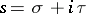, regular in a strip, and expandable into a serieswhere the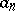are complex and theare real numbers. A real numberis called an-almost-period of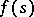if for all points of the stripthe inequality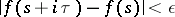holds. An almost-periodic analytic function is an analytic function that is regular in a strip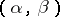and possesses a relatively-dense set of-almost-periods for every. An almost-periodic analytic function on a closed stripis defined similarly. An almost-periodic analytic function on a strip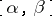is a uniformly almost-periodic function of the real variableon every straight line in the strip and it is bounded in, i.e. on any interior strip. If a function, regular in a strip, is a uniformly almost-periodic function on at least one linein the strip, then boundedness ofinimplies its almost-periodicity on the entire strip. Consequently, the theory of almost-periodic analytic functions turns out to be a theory analogous to that of almost-periodic functions of a real variable (cf. almost-periodic function). Therefore, many important results of the latter theory can be easily carried over to almost-periodic analytic functions: the uniqueness theorem, Parseval's equality, rules of operation with Dirichlet series, the approximation theorem, and several other theorems.